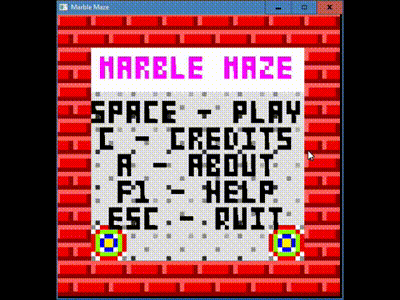-=+=- -=+=- -=+=- -=+=- -=+=- -=+=- -=+=- -=+=- -=+=- -=+=- -=+=- -=+=- -=+=- -=+=- -=+=- -=+=- -=+=- -=+=- -=+=- -=+=- -=+=- -=+=- -=+=- -=+=- -=+=- -=+=- -=+=- -=+=- -=+=- -=+=- (c) WidthPadding Industries 1987 0|658|0 -=+=- -=+=- -=+=- -=+=- -=+=- -=+=- -=+=- -=+=- -=+=- -=+=- -=+=- -=+=- -=+=- -=+=- -=+=- -=+=- -=+=- -=+=- -=+=- -=+=- -=+=- -=+=- -=+=- -=+=- -=+=- -=+=- -=+=- -=+=- -=+=- -=+=-
SoCoder -> Showcase Home -> PuzzleCreated : 12 August 2018
Edited : 12 August 2018

### Marble Maze (itch.io #lowrezjam2018)

Homepage
itch.io
ScreenshotsHere is my submission for the #lowrezjam2018 jam,
Marble Maze

Edit to add: Used BlitzMax-NG, Pyxel Edit, and TileD to create this.

## Latest UpdateSunday, 12 August 2018, 15:43
JayenkaiNice game. Simple but complex! Some nice puzzles within.Monday, 13 August 2018, 14:05
rockfordLooks good funMonday, 13 August 2018, 15:59
steve_ancellHad a little play, quite good if I may say so.Monday, 13 August 2018, 18:43Thanks for the complimentshow are the puzzles? I'm not that great at creating challenging puzzles. Difficult to get that balance between so easy that it's boring and so difficult that it's frustrating.I got to the portals levels and didnt have time to work out where the exit was